### Single Degree of Freedom Systems: Equivalent Mass and Equivalent Stiffness

We have seen that the form of the equation of motion for a simple spring{mass system is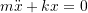Similarly, for a simple pendulum (undergoing small motions), the equation of motion can be written as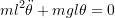which has a very similar form. In general, for any linear undamped single degree of freedom system the equation of motion can be expressed in the form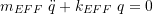whereis the chosen coordinate in the problem.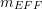and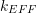are known as the effective mass and effective stiffness respectively. Note that onceare known, the natural frequency of the system can be found easily as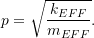### Effective Mass

So far we have foundandusing Newton’s Laws or energy methods. There are other ways to determine these quantities which may be easier in some situations. One method used to determinefor a system is to note that the kinetic energy of the SDOF system can be written as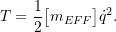where againis the chosen coordinate. Once the expression for the kinetic energy of the system is found,can be found by inspection.

#### Example

While in many situations it is appropriate to ignore the mass of a spring in a vibration analysis, any real spring will have some mass which may, in certain situations, need to be taken into account. Estimate the effect that the mass of the spring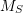has on the natural frequency of the simple spring–mass system shown below.

Begin by estimating the kinetic energy of the spring making some simplifying assumptions:

1. The mass of the spring is uniformly distributed along the length of the spring.
2. The velocity of any point on the spring is proportional to its distance from the fixed end.

### Equivalent Stiffness – Stiffness and Flexibility Approaches

To determine the effective stiffness, it can similarly be shown that the potential energy of the system is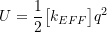so thatcan also be found by inspection once the potential energy expression has been determined. However, there are also other approaches, stiffness and flexibility methods, that can be used to determinebased on statics. These approaches are similar and are based on the idea that the force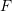applied to a system (in the positive coordinatedirection) and the resulting deflection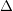(of the coordinate) are related by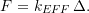In the stiffness approach, the system is given a unit displacement and the forcerequired to maintain this deformed configuration is determined through statics. The effective stiffness is then given by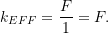In the flexibility approach, a unit load is applied to the system and the resulting deflectionis determined using statics. The effective stiffness is then given by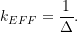In both of these approaches it is important to realize that the forceand the displacementare always measured in the positive coordinate direction. Also note that if the coordinate is a rotation(measured in radians), the same approach can be used replacing the forcewith a torque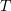. The details are summarized on the following page.

For single degree of freedom systems, the process of finding the effective stiffness can be generalized as either a stiffness or flexibility approach, both of which are static in nature and are based on the definition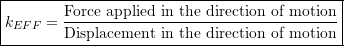##### Stiffness Approach

Linear Displacements: Displace the system such that it has a unit displacement in the positive coordinate direction. Then, using statics, determine the forcerequired in the positive coordinate direction to maintain this deflected state. Then, since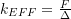and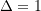,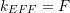Angular Displacements: Displace the system such that it has a unit angular displacement (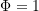) in the positive coordinate direction. Then, using statics, determine the torquerequired in the positive coordinate direction to maintain this deflected state. Then, since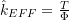and,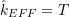##### Flexibility Approach

Linear Displacements: Apply a unit load in the positive coordinate direction and determine, through statics, the resulting deflectionin the positive coordinate direction. Then, since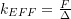and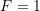,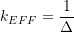Angular Displacements: Apply a unit torque in the positive coordinate direction and determine, through statics, the resulting angular deflection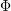in the positive coordinate direction. Then, sinceand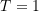,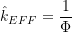##### Examples
1. Springs in Parallel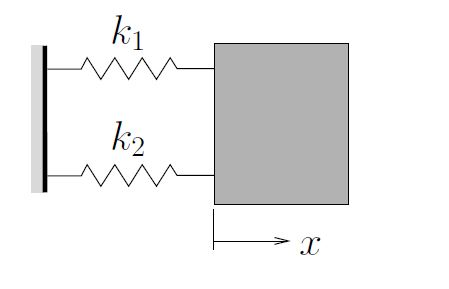2. Springs in Series3. Mass at End of Cantilever (Lateral Motions)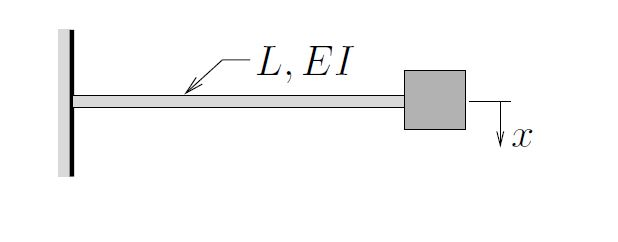4. Mass at End of Cantilever (Axial Motions)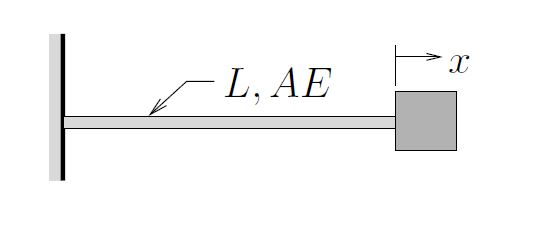5. Mass at End of Cantilever (Torsional Motions)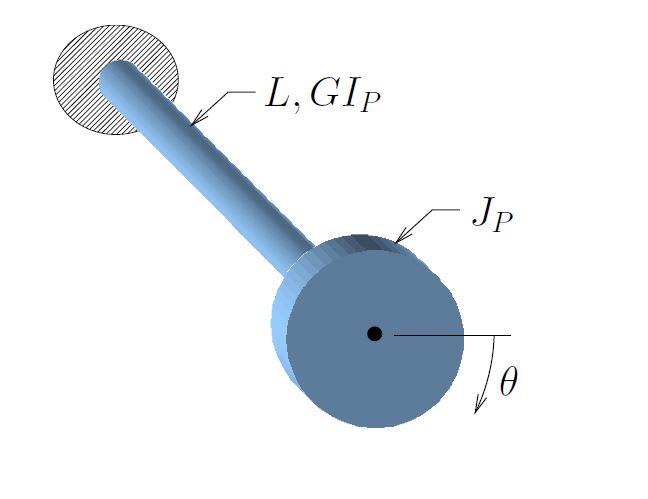Occasionally we need to modify these approaches slightly when a “unit” force or displacement would significantly change the geometry of the system resulting in a nonlinearity. In such cases we apply “small” forces or displacements instead of unit ones. Another way to think of this is to still apply a unit load, but the “unit” of the load is smaller (i.e. apply 1μN instead of 1 N). A similar approach could be taken for displacements.

#### Example 1

In the diagram below a spring supports a mass and makes an angleαwith the direction of motion. Determine the effective stiffness of the spring in this situation. Assume that only small motions occur.

#### Example 2

Determine the natural frequency for the system shown below. The double pulley set has a mass moment of inertia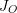about its center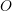. A mass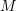is supported by a light inextensible cable.

#### Example 3

Determine the effective stiffnessof the system shown below. Neglect the mass of the bar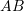.

#### Example 4

Determine the effective stiffnessof the system shown below. Neglect the mass of the bar.

#### Example 5

A block of massis supported by a cable and pulley system as shown below. Pulleys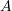and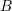are pinned to the block while pulley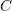is attached to a rigid support by a spring of stiffness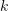. Neglecting the mass of the pulleys and treating the cable as inextensible, determine

1. the effective stiffness of the cable and pulley system,
2. the natural frequency of free vibrations.

#### Example 6

In the system shown below, a rotor with polar moment of inertia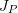is supported by a combination of shafts and gears as shown. Shafts 1 and 2 have torsional stiffnesses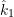and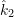respectively while gears 1 and 2 have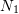and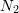teeth respectively.

Determine the effective stiffness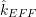for this system and the natural frequency of free vibrations. Neglect the masses of the gears.